# Calculus

##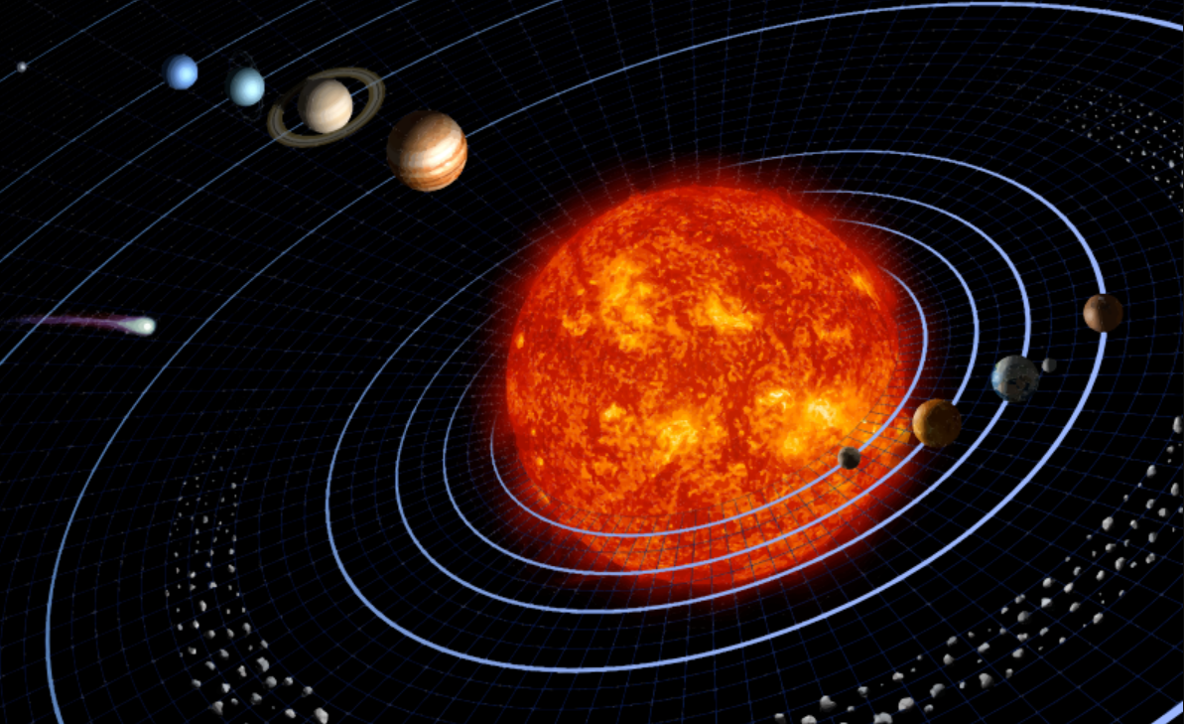Rates of change & Infinite Sums

This section is your window on to the hugely signifiant branch of mathematics called Calculus. Here the focus is very much on looking at the gradients (rates of change) of functions and how they are changing. By understanding what is happening with gradients we can understand how things are changing at instantaneous moments and works towards 'optimisation' where we look to isolate the maxima and minima of functions. Imagine this is the context of a profit function with the aim if creating the circumstances where we want to maximise the profit. Working backwards is referred to as 'anti differentiation' or 'integration' and this allows for further analysis of the area under graphs through infinite summation. For example, the gradient of a distance time graph is the speed and the area under a speed time graph is the distance travelled. It is a sophisticated development of your mathematical development and we have you covered here.

## What is in this section?

#### 5.1 & 5.3 Introduction to Calculus

This is the first of three sections on differential calculus. This is significant branch of mathematics with lots of applications. It builds very nicely on other concepts in the course and those you are likely to have covered previously. The...

#### 5.2 & 5.4 Tangents, normals and the second derivative

In this unit we look at find the equations of tangents and normals to curves, increasing and decreasing functions and the second derivative. All this builds on the fundamental notion that calculus tells us about the rate of of change of a function.

#### 5.6 & 5.7 Stationary Points and Optimisation

This is the part where we learn about how we use calculus to solve different kinds of problems. So far we have spent a lot of time learning about how calculus helps us to work out the gradient of given function for any given point. In this...

#### 5.5 & 5.8 Integration and Trapezoidal rule

In this section of the course you will learn about an area of calculus called integration. This an be considered in multiple ways, both as the inverse of differentiation (anti-differentiation) and in finding areas under curves. We will also...

## Key Questions

The following are list of key questions that you might be asking about these topics with links to the places where you can get the answers!

## What is calculus for?

Calculus is used in a huge number of situations, to find rates of change, areas, and optimise solutions.  Calculus  5.1 & 5.3 Calculus Concept

## How do you find tangents and normals

To find the tangents and normals to a curve, you must first find the gradient at that point.  5.2 & 5.4 Calculus part 2

## How do you find stationary points

Stationary points are where the gradient of a function is zero. This is a really useful property, that helps us to solve all sorts of problems.  5.6 & 5.7 Calculus Part 3

## What is the opposite of differentiation

The opposite of differentiation is integrating, and it is used all the time!  5.5 & 5.8 Integration and Trapezoidal rule

•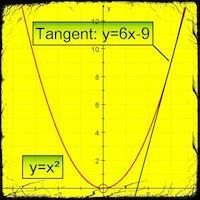#### 5.1 & 5.3 Introduction to Calculus

This is the first of three sections on differential calculus. This is significant branch of mathematics with lots of applications. It builds very nicely on other concepts in the course and those you are likely to have covered previously. The...

•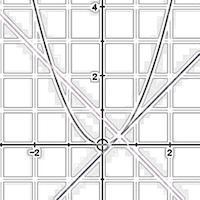#### 5.2 & 5.4 Tangents, normals and the second derivative

In this unit we look at find the equations of tangents and normals to curves, increasing and decreasing functions and the second derivative. All this builds on the fundamental notion that calculus tells us about the rate of of change of a function.

•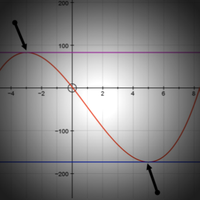#### 5.6 & 5.7 Stationary Points and Optimisation

This is the part where we learn about how we use calculus to solve different kinds of problems. So far we have spent a lot of time learning about how claculus helps us to work out the gradient of given function for any given point. In this...

•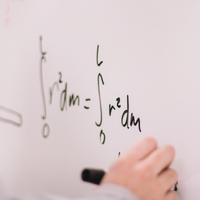#### 5.5 & 5.8 Integration and Trapezoidal rule

In this section of the course you will learn about an area of calculus called integration. This an be considered in multiple ways, both as the inverse of differentiation (anti-differentiation) and in finding areas under curves. We will also...